SNAP Library 6.0, Developer Reference  2020-12-09 16:24:20 SNAP, a general purpose, high performance system for analysis and manipulation of large networks
TNEANetMP::TNodeI Class Reference

Node iterator. Only forward iteration (operator++) is supported. More...

`#include <networkmp.h>`

Collaboration diagram for TNEANetMP::TNodeI: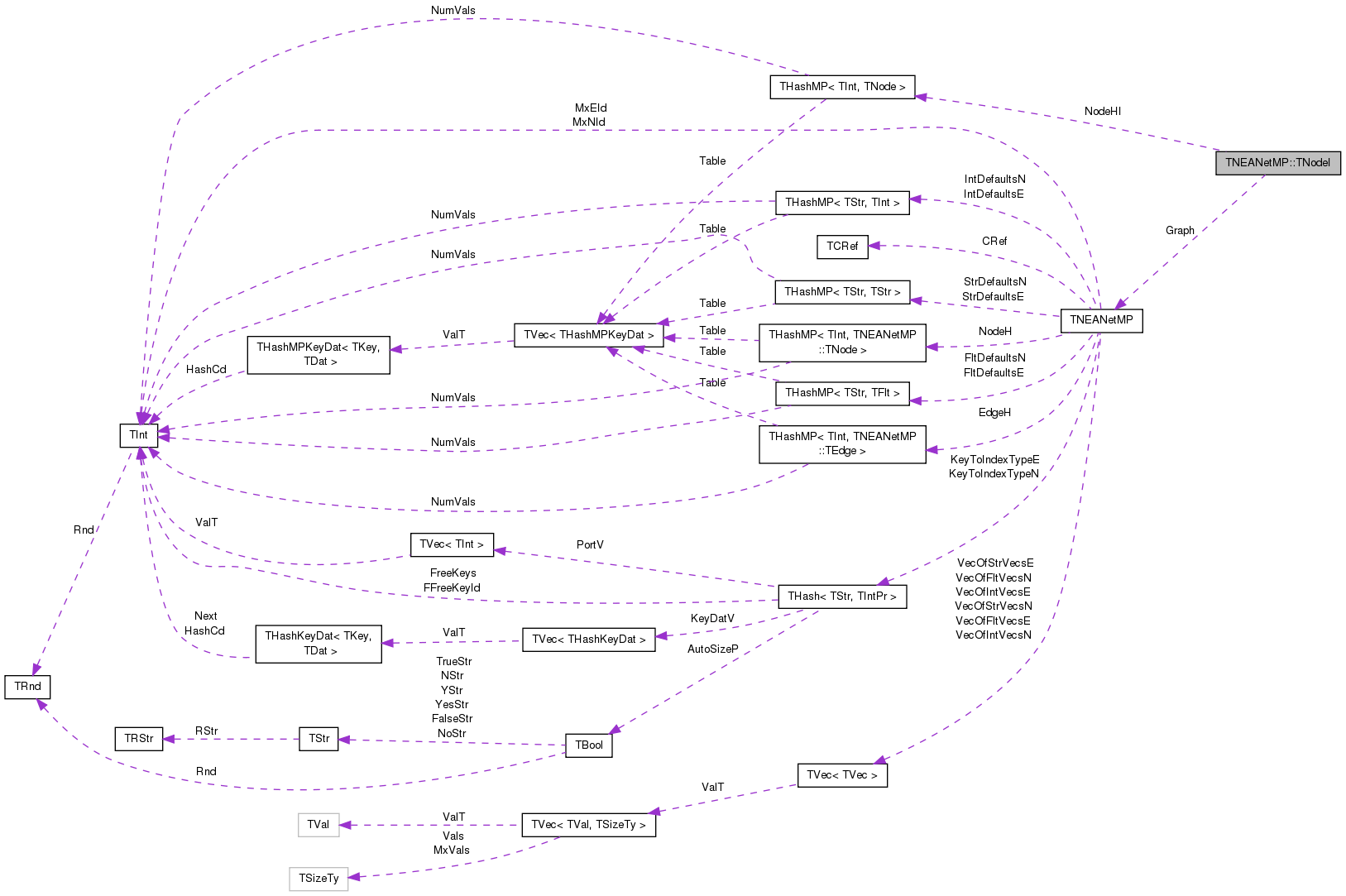[legend]

## Public Member Functions

TNodeI ()

TNodeI (const THashIter &NodeHIter, const TNEANetMP *GraphPt)

TNodeI (const TNodeI &NodeI)

TNodeIoperator= (const TNodeI &NodeI)

TNodeIoperator++ (int)
Increment iterator. More...

bool operator< (const TNodeI &NodeI) const

bool operator== (const TNodeI &NodeI) const

int GetId () const
Returns ID of the current node. More...

int GetDeg () const
Returns degree of the current node, the sum of in-degree and out-degree. More...

int GetInDeg () const
Returns in-degree of the current node. More...

int GetOutDeg () const
Returns out-degree of the current node. More...

int GetInNId (const int &EdgeN) const
Returns ID of EdgeN-th in-node (the node pointing to the current node). More...

int GetOutNId (const int &EdgeN) const
Returns ID of EdgeN-th out-node (the node the current node points to). More...

int GetNbrNId (const int &EdgeN) const
Returns ID of EdgeN-th neighboring node. More...

bool IsInNId (const int &NId) const
Tests whether node with ID NId points to the current node. More...

bool IsOutNId (const int &NId) const
Tests whether the current node points to node with ID NId. More...

bool IsNbrNId (const int &NId) const
Tests whether node with ID NId is a neighbor of the current node. More...

int GetInEId (const int &EdgeN) const
Returns ID of EdgeN-th in-edge. More...

int GetOutEId (const int &EdgeN) const
Returns ID of EdgeN-th out-edge. More...

int GetNbrEId (const int &EdgeN) const
Returns ID of EdgeN-th in or out-edge. More...

bool IsInEId (const int &EId) const
Tests whether the edge with ID EId is an in-edge of current node. More...

bool IsOutEId (const int &EId) const
Tests whether the edge with ID EId is an out-edge of current node. More...

bool IsNbrEId (const int &EId) const
Tests whether the edge with ID EId is an in or out-edge of current node. More...

void GetAttrNames (TStrV &Names) const
Gets vector of attribute names. More...

void GetAttrVal (TStrV &Val) const
Gets vector of attribute values. More...

void GetIntAttrNames (TStrV &Names) const
Gets vector of int attribute names. More...

void GetIntAttrVal (TIntV &Val) const
Gets vector of int attribute values. More...

void GetStrAttrNames (TStrV &Names) const
Gets vector of str attribute names. More...

void GetStrAttrVal (TStrV &Val) const
Gets vector of str attribute values. More...

void GetFltAttrNames (TStrV &Names) const
Gets vector of flt attribute names. More...

void GetFltAttrVal (TFltV &Val) const
Gets vector of flt attribute values. More...

## Private Types

typedef THashMP< TInt, TNode >::TIter THashIter

## Private Attributes

THashIter NodeHI

const TNEANetMPGraph

class TNEANetMP

## Detailed Description

Node iterator. Only forward iteration (operator++) is supported.

Definition at line 68 of file networkmp.h.

## Member Typedef Documentation

 typedef THashMP::TIter TNEANetMP::TNodeI::THashIter
private

Definition at line 70 of file networkmp.h.

## Constructor & Destructor Documentation

 TNEANetMP::TNodeI::TNodeI ( )
inline

Definition at line 74 of file networkmp.h.

74 : NodeHI(), Graph(NULL) { }
const TNEANetMP * Graph
Definition: networkmp.h:72
THashIter NodeHI
Definition: networkmp.h:71
 TNEANetMP::TNodeI::TNodeI ( const THashIter & NodeHIter, const TNEANetMP * GraphPt )
inline

Definition at line 75 of file networkmp.h.

75 : NodeHI(NodeHIter), Graph(GraphPt) { }
const TNEANetMP * Graph
Definition: networkmp.h:72
THashIter NodeHI
Definition: networkmp.h:71
 TNEANetMP::TNodeI::TNodeI ( const TNodeI & NodeI )
inline

Definition at line 76 of file networkmp.h.

76 : NodeHI(NodeI.NodeHI), Graph(NodeI.Graph) { }
const TNEANetMP * Graph
Definition: networkmp.h:72
THashIter NodeHI
Definition: networkmp.h:71

## Member Function Documentation

 void TNEANetMP::TNodeI::GetAttrNames ( TStrV & Names ) const
inline

Gets vector of attribute names.

Definition at line 121 of file networkmp.h.

References TNEANetMP::AttrNameNI(), and GetId().

121 { Graph->AttrNameNI(GetId(), Names); }
int GetId() const
Returns ID of the current node.
Definition: networkmp.h:83
const TNEANetMP * Graph
Definition: networkmp.h:72
void AttrNameNI(const TInt &NId, TStrV &Names) const
Returns a vector of attr names for node NId.
Definition: networkmp.h:386

Here is the call graph for this function:void TNEANetMP::TNodeI::GetAttrVal ( TStrV & Val ) const
inline

Gets vector of attribute values.

Definition at line 123 of file networkmp.h.

References TNEANetMP::AttrValueNI(), and GetId().

123 { Graph->AttrValueNI(GetId(), Val); }
int GetId() const
Returns ID of the current node.
Definition: networkmp.h:83
const TNEANetMP * Graph
Definition: networkmp.h:72
void AttrValueNI(const TInt &NId, TStrV &Values) const
Returns a vector of attr values for node NId.
Definition: networkmp.h:390

Here is the call graph for this function:int TNEANetMP::TNodeI::GetDeg ( ) const
inline

Returns degree of the current node, the sum of in-degree and out-degree.

Definition at line 85 of file networkmp.h.

85 { return NodeHI.GetDat().GetDeg(); }
THashIter NodeHI
Definition: networkmp.h:71
 void TNEANetMP::TNodeI::GetFltAttrNames ( TStrV & Names ) const
inline

Gets vector of flt attribute names.

Definition at line 133 of file networkmp.h.

References TNEANetMP::FltAttrNameNI(), and GetId().

133 { Graph->FltAttrNameNI(GetId(), Names); }
int GetId() const
Returns ID of the current node.
Definition: networkmp.h:83
void FltAttrNameNI(const TInt &NId, TStrV &Names) const
Returns a vector of int attr names for node NId.
Definition: networkmp.h:410
const TNEANetMP * Graph
Definition: networkmp.h:72

Here is the call graph for this function:void TNEANetMP::TNodeI::GetFltAttrVal ( TFltV & Val ) const
inline

Gets vector of flt attribute values.

Definition at line 135 of file networkmp.h.

References TNEANetMP::FltAttrValueNI(), and GetId().

135 { Graph->FltAttrValueNI(GetId(), Val); }
int GetId() const
Returns ID of the current node.
Definition: networkmp.h:83
const TNEANetMP * Graph
Definition: networkmp.h:72
void FltAttrValueNI(const TInt &NId, TFltV &Values) const
Returns a vector of attr values for node NId.
Definition: networkmp.h:414

Here is the call graph for this function:int TNEANetMP::TNodeI::GetId ( ) const
inline

Returns ID of the current node.

Definition at line 83 of file networkmp.h.

83 { return NodeHI.GetDat().GetId(); }
THashIter NodeHI
Definition: networkmp.h:71

Here is the caller graph for this function: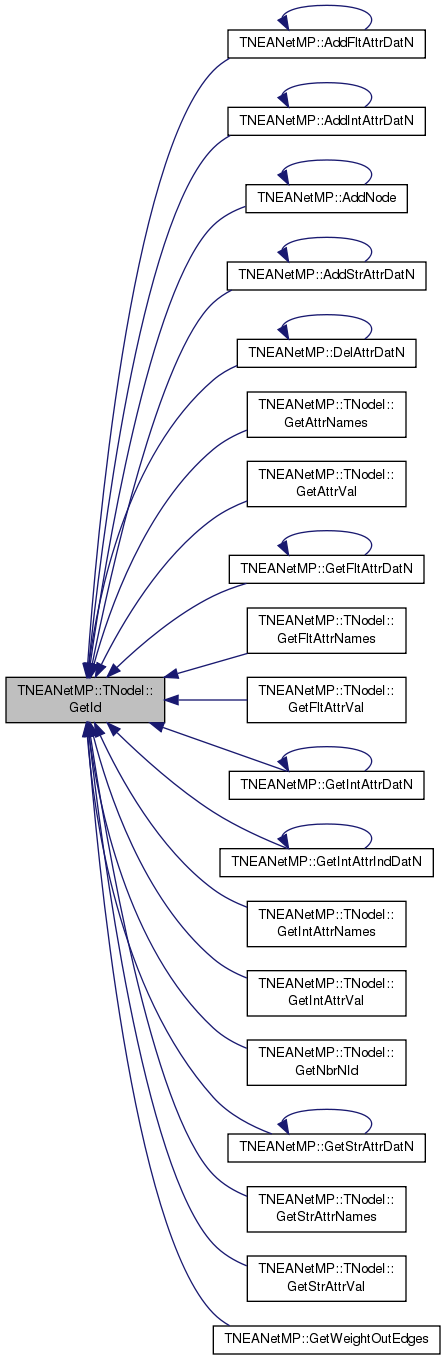int TNEANetMP::TNodeI::GetInDeg ( ) const
inline

Returns in-degree of the current node.

Definition at line 87 of file networkmp.h.

87 { return NodeHI.GetDat().GetInDeg(); }
THashIter NodeHI
Definition: networkmp.h:71
 int TNEANetMP::TNodeI::GetInEId ( const int & EdgeN ) const
inline

Returns ID of EdgeN-th in-edge.

Definition at line 109 of file networkmp.h.

109 { return NodeHI.GetDat().GetInEId(EdgeN); }
THashIter NodeHI
Definition: networkmp.h:71
 int TNEANetMP::TNodeI::GetInNId ( const int & EdgeN ) const
inline

Returns ID of EdgeN-th in-node (the node pointing to the current node).

Range of NodeN: 0 <= NodeN < GetInDeg().

Definition at line 93 of file networkmp.h.

References TNEANetMP::GetEdge().

93 { return Graph->GetEdge(NodeHI.GetDat().GetInEId(EdgeN)).GetSrcNId(); }
const TNEANetMP * Graph
Definition: networkmp.h:72
TEdge & GetEdge(const int &EId)
Definition: networkmp.h:250
THashIter NodeHI
Definition: networkmp.h:71

Here is the call graph for this function: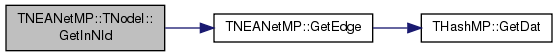void TNEANetMP::TNodeI::GetIntAttrNames ( TStrV & Names ) const
inline

Gets vector of int attribute names.

Definition at line 125 of file networkmp.h.

References GetId(), and TNEANetMP::IntAttrNameNI().

125 { Graph->IntAttrNameNI(GetId(), Names); }
int GetId() const
Returns ID of the current node.
Definition: networkmp.h:83
const TNEANetMP * Graph
Definition: networkmp.h:72
void IntAttrNameNI(const TInt &NId, TStrV &Names) const
Returns a vector of int attr names for node NId.
Definition: networkmp.h:394

Here is the call graph for this function:void TNEANetMP::TNodeI::GetIntAttrVal ( TIntV & Val ) const
inline

Gets vector of int attribute values.

Definition at line 127 of file networkmp.h.

References GetId(), and TNEANetMP::IntAttrValueNI().

127 { Graph->IntAttrValueNI(GetId(), Val); }
int GetId() const
Returns ID of the current node.
Definition: networkmp.h:83
const TNEANetMP * Graph
Definition: networkmp.h:72
void IntAttrValueNI(const TInt &NId, TIntV &Values) const
Returns a vector of attr values for node NId.
Definition: networkmp.h:398

Here is the call graph for this function: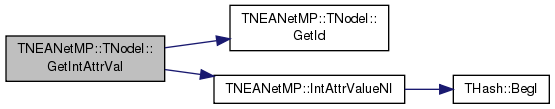int TNEANetMP::TNodeI::GetNbrEId ( const int & EdgeN ) const
inline

Returns ID of EdgeN-th in or out-edge.

Definition at line 113 of file networkmp.h.

113 { return NodeHI.GetDat().GetNbrEId(EdgeN); }
THashIter NodeHI
Definition: networkmp.h:71
 int TNEANetMP::TNodeI::GetNbrNId ( const int & EdgeN ) const
inline

Returns ID of EdgeN-th neighboring node.

Range of NodeN: 0 <= NodeN < GetNbrDeg().

Definition at line 101 of file networkmp.h.

101 { const TEdge& E = Graph->GetEdge(NodeHI.GetDat().GetNbrEId(EdgeN)); return GetId()==E.GetSrcNId() ? E.GetDstNId():E.GetSrcNId(); }
int GetId() const
Returns ID of the current node.
Definition: networkmp.h:83
const TNEANetMP * Graph
Definition: networkmp.h:72
TEdge & GetEdge(const int &EId)
Definition: networkmp.h:250
THashIter NodeHI
Definition: networkmp.h:71

Here is the call graph for this function: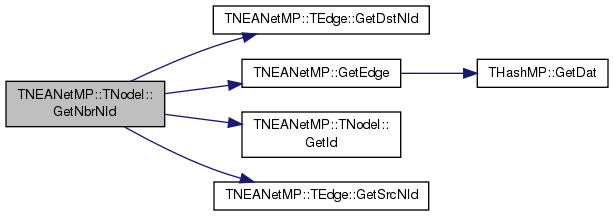int TNEANetMP::TNodeI::GetOutDeg ( ) const
inline

Returns out-degree of the current node.

Definition at line 89 of file networkmp.h.

89 { return NodeHI.GetDat().GetOutDeg(); }
THashIter NodeHI
Definition: networkmp.h:71
 int TNEANetMP::TNodeI::GetOutEId ( const int & EdgeN ) const
inline

Returns ID of EdgeN-th out-edge.

Definition at line 111 of file networkmp.h.

111 { return NodeHI.GetDat().GetOutEId(EdgeN); }
THashIter NodeHI
Definition: networkmp.h:71
 int TNEANetMP::TNodeI::GetOutNId ( const int & EdgeN ) const
inline

Returns ID of EdgeN-th out-node (the node the current node points to).

Range of NodeN: 0 <= NodeN < GetOutDeg().

Definition at line 97 of file networkmp.h.

References TNEANetMP::GetEdge().

97 { return Graph->GetEdge(NodeHI.GetDat().GetOutEId(EdgeN)).GetDstNId(); }
const TNEANetMP * Graph
Definition: networkmp.h:72
TEdge & GetEdge(const int &EId)
Definition: networkmp.h:250
THashIter NodeHI
Definition: networkmp.h:71

Here is the call graph for this function: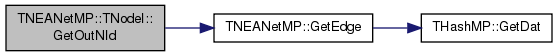void TNEANetMP::TNodeI::GetStrAttrNames ( TStrV & Names ) const
inline

Gets vector of str attribute names.

Definition at line 129 of file networkmp.h.

References GetId(), and TNEANetMP::StrAttrNameNI().

129 { Graph->StrAttrNameNI(GetId(), Names); }
int GetId() const
Returns ID of the current node.
Definition: networkmp.h:83
const TNEANetMP * Graph
Definition: networkmp.h:72
void StrAttrNameNI(const TInt &NId, TStrV &Names) const
Returns a vector of str attr names for node NId.
Definition: networkmp.h:402

Here is the call graph for this function:void TNEANetMP::TNodeI::GetStrAttrVal ( TStrV & Val ) const
inline

Gets vector of str attribute values.

Definition at line 131 of file networkmp.h.

References GetId(), and TNEANetMP::StrAttrValueNI().

131 { Graph->StrAttrValueNI(GetId(), Val); }
int GetId() const
Returns ID of the current node.
Definition: networkmp.h:83
const TNEANetMP * Graph
Definition: networkmp.h:72
void StrAttrValueNI(const TInt &NId, TStrV &Values) const
Returns a vector of attr values for node NId.
Definition: networkmp.h:406

Here is the call graph for this function:bool TNEANetMP::TNodeI::IsInEId ( const int & EId ) const
inline

Tests whether the edge with ID EId is an in-edge of current node.

Definition at line 115 of file networkmp.h.

Referenced by IsNbrEId().

115 { return NodeHI.GetDat().IsInEId(EId); }
THashIter NodeHI
Definition: networkmp.h:71

Here is the caller graph for this function: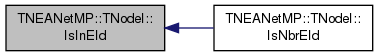bool TNEANetMP::TNodeI::IsInNId ( const int & NId ) const

Tests whether node with ID NId points to the current node.

Definition at line 9 of file networkmp.cpp.

Referenced by IsNbrNId().

9  {
10  const TNode& Node = NodeHI.GetDat();
11  for (int edge = 0; edge < Node.GetInDeg(); edge++) {
12  if (NId == Graph->GetEdge(Node.GetInEId(edge)).GetSrcNId())
13  return true;
14  }
15  return false;
16 }
const TNEANetMP * Graph
Definition: networkmp.h:72
TEdge & GetEdge(const int &EId)
Definition: networkmp.h:250
THashIter NodeHI
Definition: networkmp.h:71

Here is the call graph for this function: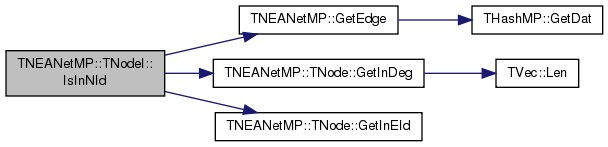Here is the caller graph for this function: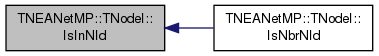bool TNEANetMP::TNodeI::IsNbrEId ( const int & EId ) const
inline

Tests whether the edge with ID EId is an in or out-edge of current node.

Definition at line 119 of file networkmp.h.

References IsInEId(), and IsOutEId().

119 { return IsInEId(EId) || IsOutEId(EId); }
bool IsOutEId(const int &EId) const
Tests whether the edge with ID EId is an out-edge of current node.
Definition: networkmp.h:117
bool IsInEId(const int &EId) const
Tests whether the edge with ID EId is an in-edge of current node.
Definition: networkmp.h:115

Here is the call graph for this function:bool TNEANetMP::TNodeI::IsNbrNId ( const int & NId ) const
inline

Tests whether node with ID NId is a neighbor of the current node.

Definition at line 107 of file networkmp.h.

References IsInNId(), and IsOutNId().

107 { return IsOutNId(NId) || IsInNId(NId); }
bool IsInNId(const int &NId) const
Tests whether node with ID NId points to the current node.
Definition: networkmp.cpp:9
bool IsOutNId(const int &NId) const
Tests whether the current node points to node with ID NId.
Definition: networkmp.cpp:18

Here is the call graph for this function: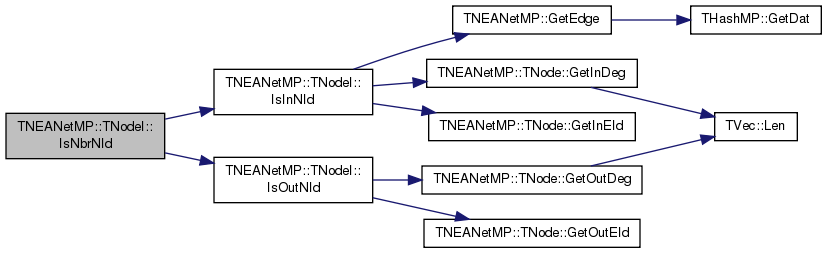bool TNEANetMP::TNodeI::IsOutEId ( const int & EId ) const
inline

Tests whether the edge with ID EId is an out-edge of current node.

Definition at line 117 of file networkmp.h.

Referenced by IsNbrEId().

117 { return NodeHI.GetDat().IsOutEId(EId); }
THashIter NodeHI
Definition: networkmp.h:71

Here is the caller graph for this function: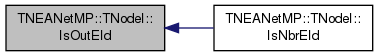bool TNEANetMP::TNodeI::IsOutNId ( const int & NId ) const

Tests whether the current node points to node with ID NId.

Definition at line 18 of file networkmp.cpp.

References edge, TNEANetMP::TNode::GetOutDeg(), and TNEANetMP::TNode::GetOutEId().

Referenced by IsNbrNId().

18  {
19  const TNode& Node = NodeHI.GetDat();
20  for (int edge = 0; edge < Node.GetOutDeg(); edge++) {
21  if (NId == Graph->GetEdge(Node.GetOutEId(edge)).GetDstNId())
22  return true;
23  }
24  return false;
25 }
const TNEANetMP * Graph
Definition: networkmp.h:72
TEdge & GetEdge(const int &EId)
Definition: networkmp.h:250
THashIter NodeHI
Definition: networkmp.h:71

Here is the call graph for this function:Here is the caller graph for this function: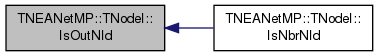TNodeI& TNEANetMP::TNodeI::operator++ ( int )
inline

Increment iterator.

Definition at line 79 of file networkmp.h.

79 { NodeHI++; return *this; }
THashIter NodeHI
Definition: networkmp.h:71
 bool TNEANetMP::TNodeI::operator< ( const TNodeI & NodeI ) const
inline

Definition at line 80 of file networkmp.h.

References NodeHI.

80 { return NodeHI < NodeI.NodeHI; }
THashIter NodeHI
Definition: networkmp.h:71
 TNodeI& TNEANetMP::TNodeI::operator= ( const TNodeI & NodeI )
inline

Definition at line 77 of file networkmp.h.

References Graph, and NodeHI.

77 { NodeHI = NodeI.NodeHI; Graph=NodeI.Graph; return *this; }
const TNEANetMP * Graph
Definition: networkmp.h:72
THashIter NodeHI
Definition: networkmp.h:71
 bool TNEANetMP::TNodeI::operator== ( const TNodeI & NodeI ) const
inline

Definition at line 81 of file networkmp.h.

References NodeHI.

81 { return NodeHI == NodeI.NodeHI; }
THashIter NodeHI
Definition: networkmp.h:71

## Friends And Related Function Documentation

 friend class TNEANetMP
friend

Definition at line 136 of file networkmp.h.

## Member Data Documentation

 const TNEANetMP* TNEANetMP::TNodeI::Graph
private

Definition at line 72 of file networkmp.h.

Referenced by IsInNId(), and operator=().

 THashIter TNEANetMP::TNodeI::NodeHI
private

Definition at line 71 of file networkmp.h.

Referenced by IsInNId(), operator<(), operator=(), and operator==().

The documentation for this class was generated from the following files: